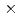Series of out-of-pile experiments to obtain the knowledge on the transient void behavior during reactivity initiated accidents are in progress at JAEA. In the present series of experiments, the transient void behavior in a test section of 22 bundle geometry under atmospheric pressure condition was measured using an impedance technique. The measuring areas and the arrangement of electrodes for the impedance technique were defined on the basis of numerical analyses and scaled model experiments. The comparison was made between the impedance and differential pressure techniques for steady boiling experiments to estimate the accuracy of the impedance technique. The impedance technique showed a good agreement with the void fraction estimated from the differential pressure. The transient void behavior in the bundle geometry was measured using the impedance technique. It was clarified that the transient void behavior depends on both the subcooling of inlet water and the heat generation rate of simulated fuel rod. Local void fraction was influenced by the ratio of flow area to heat transfer area of the simulated fuel rod.